EECS20N: Signals and Systems

# The Nyquist-Shannon Sampling Theorem

A precise statement of the Nyquist-Shannon sampling theorem is now possible. Given a continuous-time signal x with Fourier transform X where X(ω ) is zero outside the range − π /T < ω < π /T, then

x = IdealInterpolatorT (SamplerT (x)).

A formal proof of this theorem is not trivial (it was first proved by Claude Shannon of Bell Labs in the late 1940s). However, we can get much of the idea from the following argument.

Suppose now that we have a signal x with a Fourier transform X. I.e.,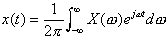.

Suppose further that it has no frequency components higher than π /T, so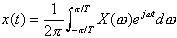.

Suppose we sample this signal with sampling interval T,

y = SamplerT (x).

Since sampling is linear (though not time invariant),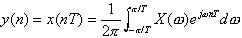Change variables, letting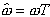,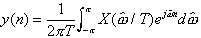because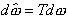. Compare this with the expression for the inverse DTFT,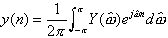This suggests that in the range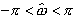,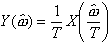.

In this range of frequencies, Y has the same shape as X, although it is scaled.

Of course, since Y is periodic with period 2π , then if we know Y in this range of frequencies, then we know it for all frequencies. We can write it as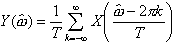.

This relationship between X and Y is illustrated below, where X is assumed to have a triangular shape.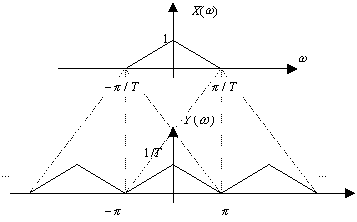Remarkably, this relationship is true for any x that is sampled with sampling interval T, regardless of whether X has frequency components higher than π /T (see box). An example is shown below where X has frequency components higher than π /T.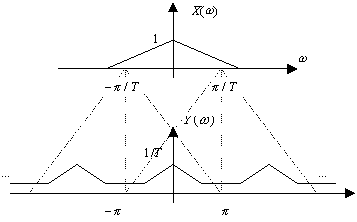Notice that in the sampled signal, the frequencies in the vicinity of π are distorted by the overlapping of frequency component above and below π /T in the original signal. This distortion is called aliasing distortion.